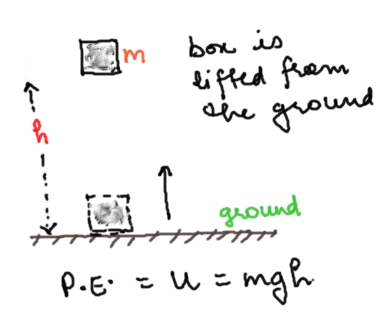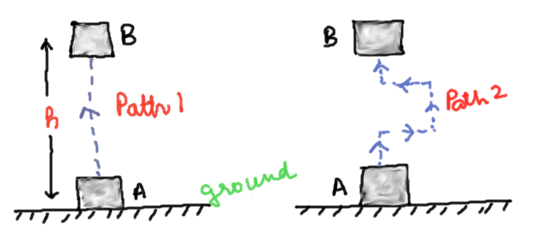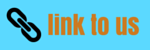# Work and energy (Class 9 CBSE)

Notes Assignment NCERT Solutions

## Energy

• We already have an idea that energy is associated closely with work and we have defined energy of a body as the capacity of the body to do work. So, an object having a capability to do work is said to possess energy.
Definition: - The capacity of an object to do work is called energy of the object.
• The object which does the work loses energy and the object on which the work is done gains energy.
• An object that possesses energy can exert a force on another object. When this happens, energy is transferred from the former to the latter.
• The energy possessed by an object is measured in terms of its capacity of doing work.
• The unit of energy is, therefore, the same as that of work, that is, joule (J). 1 J is the energy required to do 1 joule of work.
• There are various forms of energy available to us for example mechanical energy (potential energy + kinetic energy), heat energy, chemical energy, electrical energy and light energy.

### Kinetic Energy

• Kinetic energy is the energy possessed by the body by virtue of its motion
• Body moving with greater velocity would posses greater K.E in comparison of the body moving with slower velocity
• Kinetic energy of a body moving with a certain velocity is equal to the work done on it to make it acquire that velocity.
• Consider a body of mass m moving under the influence of constant force F. From Newton’s second law of motion
$F=ma$
Where a is the acceleration of the body
• If due to this acceleration a, velocity of the body increases from${v_1}$ to${v_2}$ during the displacement d then from equation of motion with constant acceleration we have
$v_2^2 - v_1^2 = 2ad$
Or,
$a = \frac{{(v_2^2 - v_1^2)}}{{2d}}$
Using this acceleration in Newton's second law of motion
we have
$F = \frac{{m\left( {v_2^2 - v_1^2} \right)}}{{2d}}$
or
$Fd = \frac{{m\left( {v_2^2 - v_1^2} \right)}}{2}$
or
$Fd = {{mv_2^2} \over 2} - {{mv_1^2} \over 2}$
We know that Fd is the work done by the force F in moving body through distance d.
• In above equation, quantity on the right hand side $\frac{1}{2}m{v^2}$ is called the kinetic energy of the body
• Thus kinetic energy possessed by an object of mass m and moving with uniform velocity v is
${E_K} = \frac{1}{2}m{v^2}$
• If the mass of the body is doubled, its kinetic energy also gets doubled and if mass of the body is halved its kinetic energy also gets halved.
• If the velocity of the body is doubled its kinetic energy becomes four times and if the velocity of the body id halved, then its kinetic energy becomes one fourth.

### Potential Energy

• Potential energy is the energy stored in the body or a system by virtue of its position in field of force or by its configuration.
• Force acting on a body or system can change its Potential Energy.
• Few examples of bodies possessing PE are given below
i) Stretched or compressed coiled spring
ii) Water stored up at a height in the Dam possess PE
iii) Any object placed above the height H from the surface of the earth posses PE
• Potential energy is denoted by letter U.
• All bodies fall towards the earth with a constant acceleration known as acceleration due to gravity.
• Consider a body of mass m placed at height h above the surface of the earth as shown below in the figure.• The force acting on this body is the gravitational pull of the earth m×g which acts in the downwards direction.
• To lift the body above the surface of the earth, we have to do work against the force of gravity. Now, Work Done= Force × distance = m×g×h
Where,
m= mass of the body;
g= acceleration due to gravity;
h= height of the body above the reference point, say the surface of earth.
• Since work done on the object is equal to $mgh$, an energy equal to $mgh$ is gained by the object. ${E_p} = mgh$
Here from the above equation we see that potential energy of any object at a height depends on the ground level or the zero level you choose.
• One important point to note hare is that work done by gravity depends on the difference in vertical heights of the initial and final positions of the object and not on the path along which the object is moved.
• To understand this point consider the figure given belowNow this figure shows the block is raised from position A to position B through two different paths. If $h$ is the height then in both the situations work done on the object is $mgh$.